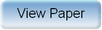Ninth International Geostatistics Congress, Oslo, Norway
June 11 – 15, 2012Session: Petroleum 1 Abstract No.: O-022 Title: Wavelet estimation in seismic convolved hidden Markov models Author(s): David Lindberg, PhD, Department of Mathematical Sciences, NTNU (NO)Henning Omre, Department of Mathematical Sciences, NTNU (NO) Abstract: Inversion of seismic AVO-data is an important part of reservoir evaluation. These data are convolved but the convolution kernel and the associated error-variances are largely unknown. We aim at estimating these model parameters without using calibration observations in wells. This constitutes the first step in socalled blind deconvolution. We solve the seismic inverse problem in a Bayesian setting and perform the associated model parameter estimation. The subsurface rock layers are represented by categorical variables, which depends on some response variables. The observations recorded appear as a convolution of these response variables. We thus assess the categorical variables' posterior distribution based on a prior distribution and a convolved likelihood distribution. Assuming that the prior model follows a Markov chain, the full model becomes a hidden Markov model. In the associated Posterior-Prior deconvolution algorithm, we approximate the convolved likelihood in order to use the recursive forward-backward algorithm. The prior and likelihood distributions are parameter dependent, and two parameter estimation approaches are discussed. Both estimation methods make use of the marginal likelihood distribution, which can be computed during the forward-backward algorithm. In two thorough test studies, we perform parameter estimation in the likelihood. Approximate posterior models, based on the respective parameter estimates, are computed by Posterior-Prior deconvolution algorithms for different orders. The signal-to-noise ratio, a ratio between the observation mean and variance, is found to be of importance. The results are generally more reliable for large values of this ratio. A more realistic seismic example is also introduced, with a more complex model description.Produced by X-CD Technologies Inc.